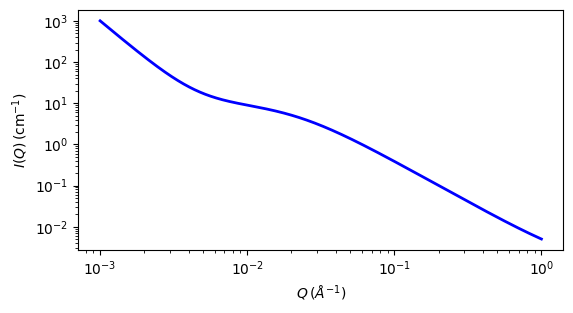# correlation_length

Calculates an empirical functional form for SAS data characterized by a low-Q signal and a high-Q signal.

Parameter

Description

Units

Default value

scale

Scale factor or Volume fraction

None

1

background

Source background

cm-1

0.001

lorentz_scale

Lorentzian Scaling Factor

None

10

porod_scale

Porod Scaling Factor

None

1e-06

cor_length

Correlation length, xi, in Lorentzian

50

porod_exp

Porod Exponent, n, in q^-n

None

3

lorentz_exp

Lorentzian Exponent, m, in 1/( 1 + (q.xi)^m)

None

2

The returned value is scaled to units of cm-1 sr-1, absolute scale.

Definition

The scattering intensity I(q) is calculated as

$I(Q) = \frac{A}{Q^n} + \frac{C}{1 + (Q\xi)^m} + \text{background}$

The first term describes Porod scattering from clusters (exponent = $$n$$) and the second term is a Lorentzian function describing scattering from polymer chains (exponent = $$m$$). This second term characterizes the polymer/solvent interactions and therefore the thermodynamics. The two multiplicative factors $$A$$ and $$C$$, and the two exponents $$n$$ and $$m$$ are used as fitting parameters. (Respectively porod_scale, lorentz_scale, porod_exp and lorentz_exp in the parameter list.) The remaining parameter $$\xi$$ (cor_length in the parameter list) is a correlation length for the polymer chains. Note that when $$m=2$$ this functional form becomes the familiar Lorentzian function. Some interpretation of the values of $$A$$ and $$C$$ may be possible depending on the values of $$m$$ and $$n$$.

For 2D data: The 2D scattering intensity is calculated in the same way as 1D, where the q vector is defined as

$q = \sqrt{q_x^2 + q_y^2}$Fig. 99 1D plot corresponding to the default parameters of the model.

Source

correlation_length.py

References

1. B Hammouda, D L Ho and S R Kline, Insight into Clustering in Poly(ethylene oxide) Solutions, Macromolecules, 37 (2004) 6932-6937

Authorship and Verification

• Author: NIST IGOR/DANSE Date: pre 2010